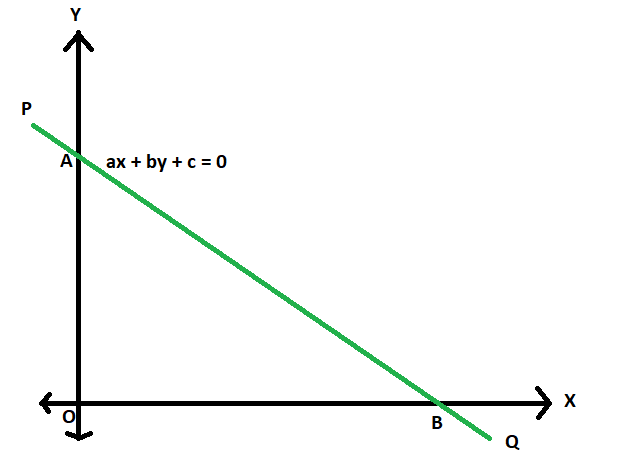GeeksforGeeks App
Open AppBrowser
Continue

# Area of triangle formed by the axes of co-ordinates and a given straight line

Given a straight line with equation coefficients as a, b & c(ax + by + c = 0), the task is to find the area of the triangle formed by the axes of co-ordinates and this straight line.
Examples:

```Input: a = -2, b = 4, c = 3
Output: 0.5625

Input: a = 4, b = 3, c = 12
Output: 6```Approach

1. Let PQ be the straight line having AB, the line segment between the axes.
The equation is,
ax + by + c = 0

2. so, in intercept form it can be expressed as,
x/(-c/a) + y/(-c/b) = 1

3. So, the x-intercept = -c/a
the y-intercept = -c/b

4. So, it is very clear now the base of the triangle AOB will be -c/a
and the base of the triangle AOB will be -c/b

5. So, area of the triangleBelow is the implementation of the above approach:

## C++

 `// C++ program area of triangle``// formed by the axes of co-ordinates``// and a given straight line` `#include ``using` `namespace` `std;` `// Function to find area``double` `area(``double` `a, ``double` `b, ``double` `c)``{``    ``double` `d = ``fabs``((c * c) / (2 * a * b));``    ``return` `d;``}` `// Driver code``int` `main()``{``    ``double` `a = -2, b = 4, c = 3;``    ``cout << area(a, b, c);``    ``return` `0;``}`

## Java

 `// Java program area of triangle``// formed by the axes of co-ordinates``// and a given straight line` `import` `java.io.*;` `class` `GFG``{` `// Function to find area``static` `double` `area(``double` `a, ``double` `b, ``double` `c)``{``    ``double` `d = Math.abs((c * c) / (``2` `* a * b));``    ``return` `d;``}` `// Driver code``public` `static` `void` `main (String[] args)``{``    ` `    ``double` `a = -``2``, b = ``4``, c = ``3``;``    ``System.out.println(area(a, b, c));``}``}` `// This code is contributed by ajit.`

## Python3

 `# Python3 program area of triangle``# formed by the axes of co-ordinates``# and a given straight line` `# Function to find area``def` `area(a, b, c):` `    ``d ``=` `abs``((c ``*` `c) ``/` `(``2` `*` `a ``*` `b))``    ``return` `d` `# Driver code``a ``=` `-``2``b ``=` `4``c ``=` `3``print``(area(a, b, c))` `# This code is contributed``# by mohit kumar`

## C#

 `// C# program area of triangle``// formed by the axes of co-ordinates``// and a given straight line``using` `System;` `class` `GFG``{``    ` `// Function to find area``static` `double` `area(``double` `a, ``double` `b, ``double` `c)``{``    ``double` `d = Math.Abs((c * c) / (2 * a * b));``    ``return` `d;``}` `// Driver code``static` `public` `void` `Main ()``{``    ` `    ``double` `a = -2, b = 4, c = 3;``    ``Console.WriteLine (area(a, b, c));``}``}` `// This code is contributed by akt_mit.`

## PHP

 ``

## Javascript

 ``

Output:

`0.5625`

Time Complexity: O(1)

Auxiliary Space: O(1)

My Personal Notes arrow_drop_up